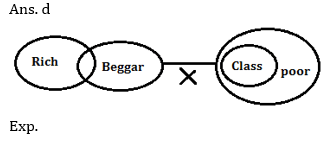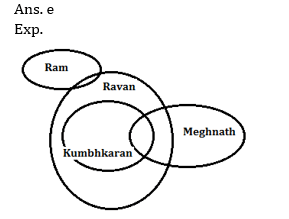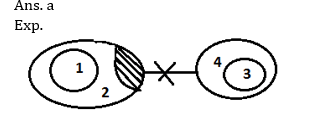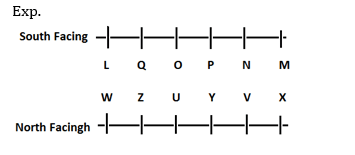# RRB PO Prelims Reasoning Ability Quiz – 22

## RRB PO Prelims Reasoning Ability Quiz

Reasoning Ability plays a very crucial role in every competitive examination. With consistent practice, candidates can ace this section in examination. In this article, we bring to you RRB PO Reasoning Ability Quiz to boost your preparation. This RRB PO Reasoning Ability Quiz contains various types of questions ranging from easy to difficult level. This RRB PO Reasoning Ability Quiz is absolutely FREE. Candidates will be provided with a detailed explanation of each question in this RRB PO Reasoning Ability Quiz. Question given in this RRB PO Reasoning Ability Quiz are based on the latest or updated exam pattern. In order to be able to answer questions quickly and efficiently in upcoming exams, aspirants must practice this RRB PO Reasoning Ability Quiz.

Directions (1-5): In each question below are given two/three statements followed by two conclusions numbered I and II. You have to take the given statements to be true even if they seem to be at variance with commonly known facts. Read all the conclusions and then decide which of the given conclusions logically follows from the given statements, disregarding commonly known facts. Give answer

1. Statements: No beggar is a poor.
Some rich are beggars. All classes are poors.
Conclusions: I. All rich being poors is a possibility.
II. All beggars being classes is a possibility.
(a) if only conclusion I follows.
(b) if only conclusion II follows.
(c) if either conclusion I or II follows.
(d) if neither conclusion I nor II follows.
(e) if both conclusions I and II follow.2. Statements: No beggar is a poor.
Some rich are beggars. All classes are poors.
Conclusions: I.Some poors are not rich.
II. No class is a beggar.
(a) if only conclusion I follows.
(b) if only conclusion II follows.
(c) if either conclusion I or II follows.
(d) if neither conclusion I nor II follows.
(e) if both conclusions I and II follow.3. Statements: Some Ram are Ravan.
Some Meghnath are Kumbhkarn.
All Kumbhkarn are Ravan.
Conclusions:
I. All Ram being Kumbhkarn is a possibility.
II. Some Ravan are not Meghnath is a possibility.
(a) if only conclusion I follows.
(b) if only conclusion II follows.
(c) if either conclusion I or II follows.
(d) if neither conclusion I nor II follows.
(e) if both conclusions I and II follow.4. Statements: All One are Two.
All Three are Four. Some Two are not four.
Conclusions: I. Some two are not three.
II. No One is a Four.
(a) if only conclusion I follows.
(b) if only conclusion II follows.
(c) if either conclusion I or II follows.
(d) if neither conclusion I nor II follows.
(e) if both conclusions I and II follow.5. Statements: No 90s are 80s.
Some 90s are 40s. All 80s are 70s.
Conclusions: I. All 80s being 40s is a possibility.
II. Some 70s are not 90s.
(a) if only conclusion I follows.
(b) if only conclusion II follows.
(c) if either conclusion I or II follows.
(d) if neither conclusion I nor II follows.
(e) if both conclusions I and II follow.Directions (6-10): Study the following information to answer the given questions:
Twelve people are sitting in two parallel rows containing six people each, in such a way that there is an equal distance between adjacent persons. In row-1 L, M, N, O, P and Q are seated and all of them are facing South. In row-2 U, V, W, X, Y and Z are seated and all of them are facing North. Therefore, in the given seating arrangement each member seated in a row faces another member of the other row.
O sits third to right of M. Either O or M sits at an extreme end of the line. The one who faces M sits second to right of Y. Two people sit between V and Z. Neither V nor Z sits at an extreme end of the line. The immediate neighbor of V faces the person who sits third to left of L. N and P are immediate neighbors of each other. W sits second to the left of U. P does not face the immediate neighbor of X.

6. Who amongst the following sit at extreme ends of the rows ?
(a) O, X
(b) Q, U
(c) Q, W
(d) L, X
(e) M, Z

Ans. d7. How many persons are seated between Q and N?
(a) One
(b) Two
(c) Three
(d) Four
(e) None

Ans. b8. L is related to U in the same way as O is related to V based on the given arrangement. To which of the following is P related to, following the same pattern?
(a) W
(b) X
(c) Y
(d) Z
(e) Cannot be determined

Ans. b9. Which of the following is true regarding P ?
(a) Z faces P
(b) M is an immediate neighbor of P
(c) Z faces the one who is second to right of P
(d) P sits at one of the extreme ends of the line
(e) M sits second to the right of P

Ans. c10. How many persons are seated between U and X ?
(a) One
(b) Two
(c) Three
(d) Four
(e) None

Ans. b###Click to Buy Bank MahaCombo PackageRecommended PDF’s for:

#### Most important PDF’s for Bank, SSC, Railway and Other Government Exam : Download PDF Now

AATMA-NIRBHAR Series- Static GK/Awareness Practice Ebook PDF Get PDF here
The Banking Awareness 500 MCQs E-book| Bilingual (Hindi + English) Get PDF here
AATMA-NIRBHAR Series- Banking Awareness Practice Ebook PDF Get PDF here
Computer Awareness Capsule 2.O Get PDF here
AATMA-NIRBHAR Series Quantitative Aptitude Topic-Wise PDF Get PDF here
AATMA-NIRBHAR Series Reasoning Topic-Wise PDF Get PDF Here
Memory Based Puzzle E-book | 2016-19 Exams Covered Get PDF here
Caselet Data Interpretation 200 Questions Get PDF here
Puzzle & Seating Arrangement E-Book for BANK PO MAINS (Vol-1) Get PDF here
ARITHMETIC DATA INTERPRETATION 2.O E-book Get PDF here
3